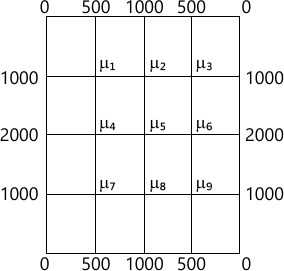MORE IN Engineering Mathematics 3
VTU Computer Science (Semester 3)
Engineering Mathematics 3
June 2014
Total marks: --
Total time: --
INSTRUCTIONS
(1) Assume appropriate data and state your reasons
(2) Marks are given to the right of every question
(3) Draw neat diagrams wherever necessary

1 (a) Find Fourier series of f(x) = 2?x-x2 in [0, 2?]. Hence deduce $\sum^\infty_1 \dfrac {1}{(2n-1)^2}=\dfrac {\pi^2}{8}$ sketch the graph of f(x).
7 M
1 (b) Find Fourier cosin series of $f(x)=\sin \left ( \dfrac {m \pi}{l} \right )x$ where m is positive integer.
6 M
1 (c) Following table gives current (A) over period (T):
 A (amp) 1.98 1.30 1.05 1.30 -0.88 -0.25 1.98 t (sec) 0 T/6 T/3 T/2 2T/3 5T/6 T

Find amplitude of first harmonic.
7 M

2 (a) Find Fourier transformation of e-a2x2 (-? < x < ?) hence show that e -x2/2 is self reciprocal.
7 M
2 (b) Find Fourier cosin and sine transformation of $f(x)= \left\{\begin{matrix} x&0 6 M 2 (c) Solve integral equation \[ \int^\infty _0f(x)\cos sxdx=\left\{\begin{matrix} 1-s&0 7 M 3 (a) Find the various possible solution of one dimesional wave equation \[ \dfrac {\partial^2u}{\partial t^2}=C^2\dfrac {\partial^2 u}{\partial x^3}$ by separable variable method.
7 M
3 (b) Obtain solution of heat equation $\dfrac {\partial u} {\partial t}=c^2\dfrac {\partial^2 u}{\partial x^2}$ subject to condition u(0,t)=0, u(l,t)=0, u(x,0)=f(x)
6 M
3 (c) Solve Laplace equation $\dfrac {\partial^2 u}{\partial x^2}+\dfrac {\partial ^2 u}{\partial y^2}=0$ subjects to condition u(0,y)=u(l,y)=u(x,0)=0; $u(x,a)=\sin \left ( \dfrac {\pi x}{l} \right )$
7 M

4 (a) The revolution (r) and time (t) are related by quadratic polynomial r=at2+bt+c. Estimate number revolution for time 3.5 units. Given
 Revolution 5 10 15 20 25 30 35 Time 1.2 1.6 1.9 2.1 2.4 2.6 3
7 M
4 (b) Solve by graphical method, Minimize Z=20x1+10x2 under the constraints 2x1+x2?0; x1+2x2?40; 3x1+x2?0; 4x1+3x2?60; x1, x2?0
6 M
4 (c) A company produces 3 items A, B, C. Each unit of A requires 8 minutes, 4 minutes and 2 minutes of producing time on machine M1, M2 and M3 respectively. Similarly B requires 2, 3, 0 and C requires 3, 0, 1 minutes of machine M1 M2 and M3. Profit per unit of A, B and C are Rs. 20, Rs 6 and Rs.8 respectively. For maximum profit. Given machine M1, M2, M3 are available for 250, 100 and 60 minutes per day.
7 M

5 (a) BY relaxation method, solve -x+6y+27z=85, 54x+y+z=110, 2x+15y+6z=72
7 M
5 (b) Using Newton Raphson method derive the iteration formula to find the value reciprocal of positive number. Hence use to find 1/c upto 4 decimals.
6 M
5 (c) Using power relay method find numerical largest eigen value and corresponding eigen vector for $\begin{bmatrix}10 &2 &1 \\2 &10 &1 \\2 &1 &10 \end{bmatrix}$ using (I, I, 0)T as initial vector. Carry out 10 iterations.
7 M

6 (a) Fit interpolating polynomial for f(x) using divided difference formula and hence evaluate f(z), given f(0)=-5, f(1)=-14, f(4)=-125, f(8)=-21, f(10)=335.
7 M
6 (b) Estimate t when f(t)=85, using inverse intepolation formula given:
 t 2 5 8 14 f(t) 94.8 87.9 81.3 68.7
6 M
6 (c) A solid of revolution is formed by rotating about x-axis the area between x-axis, line x-0, x-1 and curve through the points with the following co-ordinates.
 x 0 1/6 2/6 3/6 4/6 5/6 1 y 0.1 0.8982 0.9018 0.9589 0.9432 0.9001 0.8415

by Simpson's 3/8th rule find the volume of solid formed.
7 M

7 (a) Using the Schmidt two-level point formula solve $\dfrac {\partial^2 u}{\partial x^2}=\dfrac {\partial u}{\partial t}$ under the conditions u(0,t)=u(1,t)=0; t?0; u(1,0) = sin ? x 0< x < 1, take $h=\dfrac {h}{1}\alpha - \dfrac {1}{6}$ Carry out 3 steps in time level.
7 M
7 (b) Solve the wave equation $\dfrac {\partial^2u}{\partial t^2}=4\dfrac {\partial^2u}{\partial x^2}$ subject to u(0,t)=u(4,t)=ut(x,0)=0, u(x,0)=x(4-x) take h=1 k=0.5
6 M
7 (c) $Solve \ \dfrac {\partial^2 u}{\partial x^2}+\dfrac {\partial^2 u}{\partial y^2}=0$ in the square mesh. Carry out 2 iterations.7 M

8 (a) State and prove recurrence relation of f-transformation hence find ZT(n), ZT(n2)
7 M
8 (b) Find ZT [ en? cosh n? - sin (nA+?)+n]
6 M
8 (c) Solve difference equation un+2+6un+1 +9un=n2n given u0=u1=0
7 M

More question papers from Engineering Mathematics 3